# Numeral and Number Systems. Origin and Development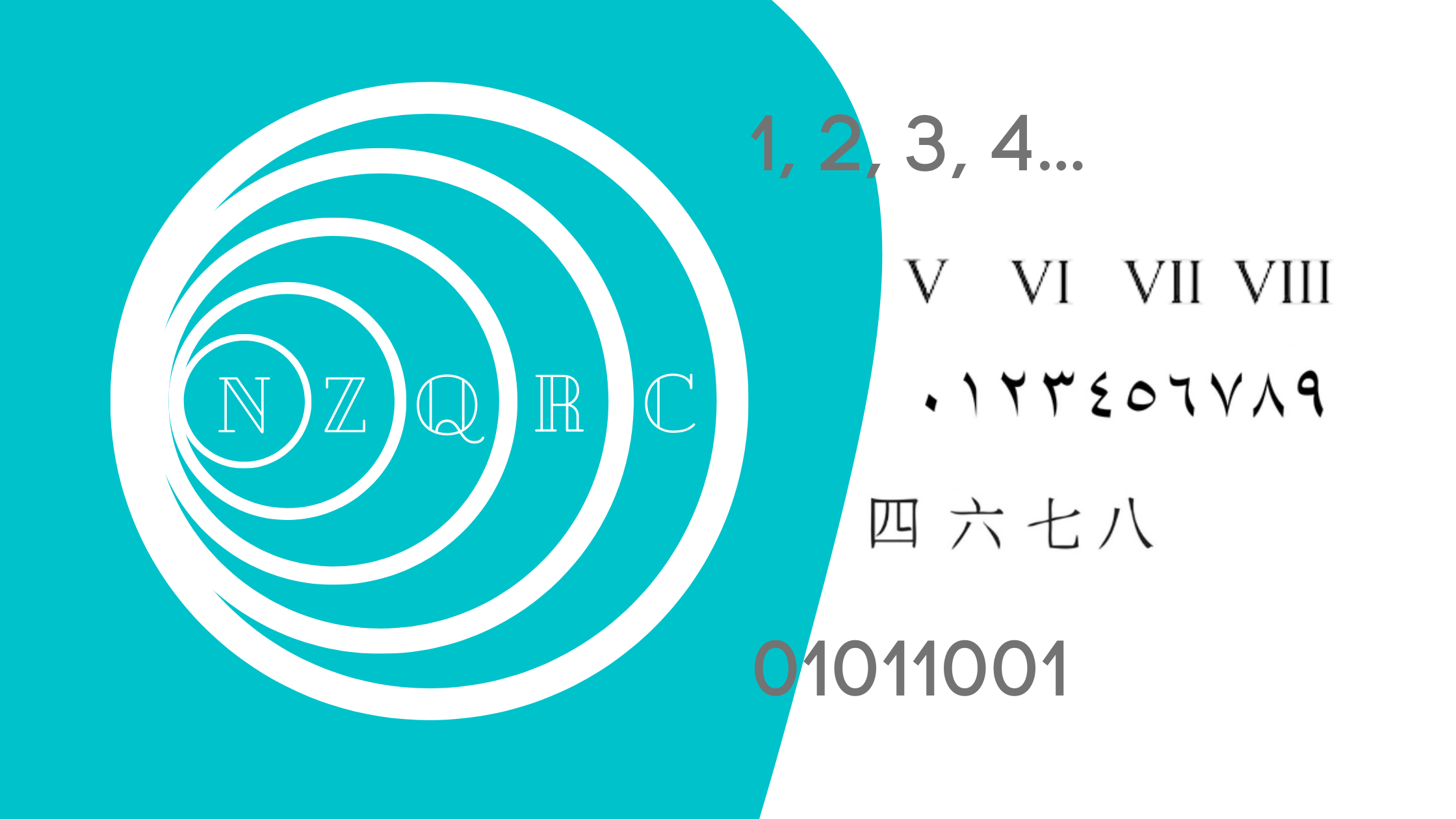A number is an abstract concept used to compute or measure something.

A numeral is a symbol representing a number.

A number system is a set of numbers sharing the same characteristics.

A numeral system is a combination of specific numerals.

People have been trying to store and pass the information on as soon as they learned how to communicate.

The first attempts to depict numbers were done using the images of sticks or stones – mostly to count the number of items.

As civilization advanced, the need to develop numeral and number systems that would allow people to complete more complex mathematical operations grew. With the implementation of taxation systems, increased trade, evolving construction needs, interest in sciences, mathematicians were looking for more efficient ways of representing numbers.

## Number Bases and Number Groups

Counting up to 10 (based on the number of fingers on one’s hand) eventually became not enough and people had to think of a way of representing larger numbers using symbols.

Thus, the number bases and number groups emerged.

10 was a popular base (however, Babylonians, for example, used base-sixty and Mayans used base-twenty), because it was easy to count on fingers and count by 10s.

Other numbers were also used to represent groups of numbers (a dozen, for example).

The symbols representing ones and tens first appeared in Egypt and Mesopotamia around 3400-3000 BC.

Simple grouping numeral systems were used to depict large numbers and mathematical expressions involving more than one arithmetic operation.

Egyptians repeated the symbols up to 9, with a special symbol for 10.

Greeks and Romans had a similar way of representing large numbers and performing arithmetic operations.

Roman numerals were popular for almost 2000 years.

The Roman numerals system was a convenient system based on letters: I, V, X, L, C, D and M.

The system first emerged between 900 and 800 B.C and is still used in some contexts today.

## Modern Numeral System

The decimal numeral system that we use today originated in India and was further developed in Persia.

The Chinese system was also a decimal system, where counting was based on ten numerals represented by symbols. It had special characters to represent ten, a hundred, a thousand, ten thousand, as well as other multiples of ten.

The modern numerals system is called Hindu-Arabic: 1, 2, 3, 4, 5, 6, 7, 8, 9 and 0.

The system is positional, which means that the position of a symbol determines the place value of that symbol within the number (235 is two hundred + thirty + five).

Around 1500 BC – 500, Indians were really interested in Astronomy and thus, the calculations involved very large numbers. They expressed these numbers using a place-value notation, giving names for the powers of 10.

The full system was first described outside India in Al-Khwarizmi’s On the Calculation with Hindu Numerals ( in 825).

The earliest European manuscript, Codex Vigilanus, containing these numerals was written in Spain in 976. An Italian mathematician, Fibonacci, further popularized the system in Europe.

## Number Systems

Numbers are classified into number systems based on their characteristics and properties.

There are 5 main number systems that we use to classify numbers:

• Natural Numbers
• Integers
• Rational Numbers
• Real Numbers
• Complex (Imaginary) Numbers

Natural numbers – positive integers that we use to count.

The symbol for the set of all natural numbers is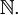Integers – a combination of a zero, negative and positive whole numbers. Zero is neither positive nor negative. Negative numbers are numbers that are less than zero. We use them to depict opposites, deficits, etc.

The abstract concept of negative numbers was described around 100 B.C.. – 50 B.C. in the Chinese ”Nine Chapters on the Mathematical Art” (Jiu-zhang Suanshu) which provided explanations on how to find areas of figures where red lines were used for positive coefficients and black were used for negative coefficients.

Greek mathematician Diophantus described integers and integer equations in his work Arithmetica.

The symbol for the set of all integers is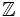(German Zahl, plural Zahlen – meaning “numbers”).

Rational numbers – numbers that can be expressed as fractions with an integer numerator and a non-zero natural number denominator. The denominator cannot be 0 since we cannot divide by 0. But a value of the whole fraction can equal 0.

Fractions can be positive and negative.

Integers are part of the set of all rational numbers, since every integer can be given as a fraction with a denominator 1.

Rational numbers could be found in the texts of Ancient Egypt, describing how to convert fractions. Indian and Greek mathematicians studied rational numbers as part of the number theory.

The symbol for the set of all rational numbers is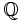(meaning “quotient” – the outcome of the division).

Irrational numbers are numbers that cannot be expressed as repeating, terminating decimals or as a ratio of two integers. Two special examples of irrational numbers are numbers 𝚎 and 𝛑 .

The need for understanding and considering irrational numbers was established around 500 BC by a Greek mathematician Pythagoras.

These numbers do not have their own set symbol.

Real numbers – all of the rational and irrational numbers ( (-) – from negative to positive infinity). On a number line real numbers fill up all the space.

In the 17th century, Rene Decartes introduced the term “real” to describe roots of a polynomial (distinguishing them from imaginary numbers). Although with the development of Calculus real numbers sets and the concept of infinity were widely used, real numbers were for the first time formally defined in 1871 by Georg Cantor.

The symbol for the real numbers is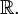Complex (imaginary) numbers result from taking a square root of a negative number. The resulting number is denoted by i, a symbol assigned by Leonhard Euler.

The symbol for the real numbers is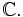Every preceding set of numbers is a subset for the subsequent number system.

## Binary Number System

A Binary number system is the simplest of all positional systems and is widely used in Computer Science (computers use the binary number system to manipulate and store all of their data including numbers, words, videos, graphics, and music).

The base of the binary system is 2 and only two numbers are used: 0 and 1.

Gottfried Leibniz, the co-creator of Calculus, first published his invention of the binary system in the 17th century.

In a binary number system the first 10 numbers would be represented as:

0, 1, 10, 11, 100, 101, 110, 111, 1000, 1001, and 1010

We can convert binary numbers into decimal numbers.

To convert 10101 from binary to decimal system we would do the following (a subscripts represents the base – 2 for binary and 10 for decimal):

Converting from binary to decimal, a binary number for would be

Also check out:

Grades 5 – 11 Free Math Lessons and Practice

Grades 5-12 Free Online Math Quizzes

0